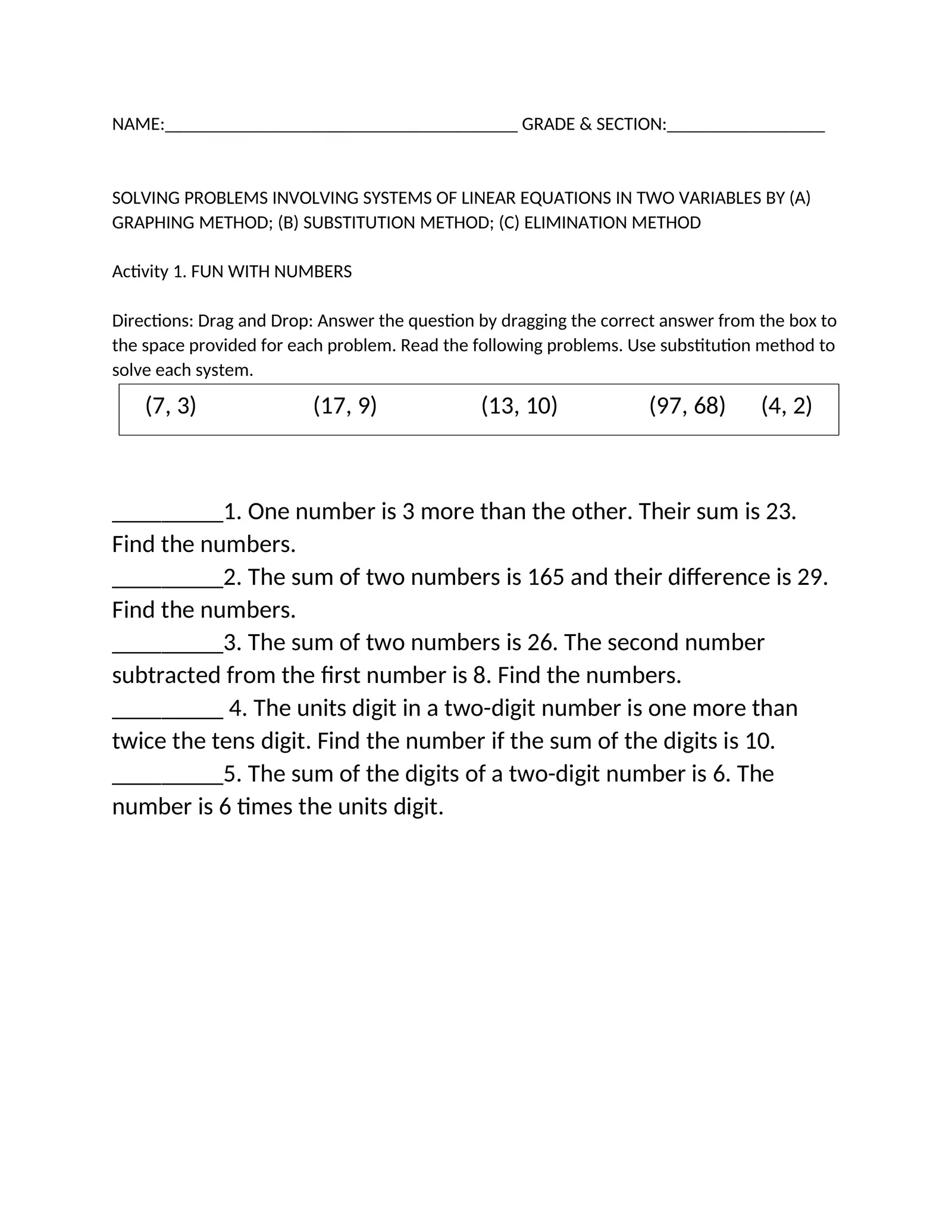# Act

Created

NAME:______________________________________ GRADE & SECTION:_________________ SOLVING PROBLEMS INVOLVING SYSTEMS OF LINEAR EQUATIONS IN TWO VARIABLES BY (A) GRAPHING METHOD; (B) SUBSTITUTION METHOD; (C) ELIMINATION METHOD Activity 1. FUN WITH NUMBERS Directions: Drag and Drop: Answer the question by dragging the correct answer from the box to the space provided for each problem. Read the following problems. Use substitution method to solve each system. (7, 3) (17, 9) (13, 10) (97, 68) (4, 2) _________1. One number is 3 more than the other. Their sum is 23. Find the numbers. _________2. The sum of two numbers is 165 and their difference is 29. Find the numbers. _________3. The sum of two numbers is 26. The second number subtracted from the first number is 8. Find the numbers. _________ 4. The units digit in a two-digit number is one more than twice the tens digit. Find the number if the sum of the digits is 10. _________5. The sum of the digits of a two-digit number is 6. The number is 6 times the units digit.